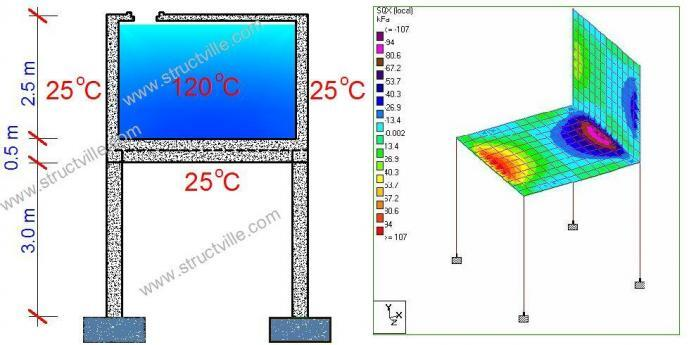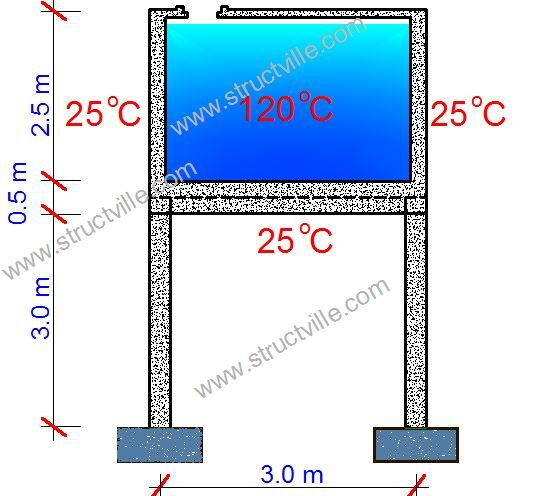# Evaluation of effects of temperature difference in storage tanks using Staad ProIn some factories and industries, tanks are used for the storage of hot liquids which are used in production. In such scenarios, the temperature inside the tank and the temperature in the surrounding may not be the same. It is well known that internal forces are induced in statically indeterminate structures when there is temperature difference as the elements undergo differential thermal expansion/contraction. For simple frames, the internal forces due to temperature difference can be easily obtained using the force method of structural analysis. But for more complex structures like combination of beams and plates, software like Staad Pro can be used for evaluation of temperature difference.

For example, let us consider the reinforced concrete tank with the dimensions shown in Figure 1;Fig 1: Structural scheme of water tank subjected to temperature difference

Dimensions of columns = 300 x 300 mm
Dimension of beams = 300 x 500 mm
Height of column above ground level = 3 m
Length of tank = Width of tank = 3 m (centre to centre)
Height of tank = 2.5 m (centre to centre)
Thickness of tank walls and base = 250 mm
Support condition = Fixed
Temperature inside the tank = 120 oC
Temperature outside the tank = 25 oC
Maximum hydrostatic pressure from the liquid stored = 25 kPa
Modulus of elasticity of concrete = 2.8 x 107 kN/m2
Coefficient of expansion of concrete = 1.0 x 10-5 /oC

The tank has been modelled on Staad Pro (see Figure 2) using the parameters defined above.

The walls of the tank were subjected to a triangular hydrostatic pressure distribution of 25 kPa. You can check how apply hydrostatic loads on Staad Pro here. The temperature difference action applied to the the tank is shown below.

Temperature change for axial elongation = Average temperature = (25 + 120)/2 = 72.5 oC
Temperature difference = 25 – 120 = -95 oC

The application on Staad Pro is shown in Figure 3.